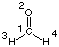## Gaussian Input Files

The following is a typical Gaussian input file, using a single point energy calculation on formaldehyde (HF/6-31G(d) level) as an example:
 ```%mem=6000000 %chk=/scratch/test1.chk #P HF/6-31G(d) scf=tight test1 HF/6-31G(d) sp formaldehyde 0 1 C1 O2 1 r2 H3 1 r3 2 a3 H4 1 r4 2 a4 3 d4 r2=1.20 r3=1.0 r4=1.0 a3=120. a4=120. d4=180. ```The first line of the input file:

```
%mem=6000000

```
specifies how much memory is available for this calculation (in words). The default value (if nothing is specified) amounts to 6000000 words (corresponding to 48MB on a 32bit operating system). This information can also be specified with
```
%mem=48MB

```
The second line of the input file:
```
%chk=/scratch/test1.chk

```
defines a binary "checkpoint" file to which important results are written. If no file name is supplied in the input file, the checkpoint files are generated during program execution, but deleted on successful termination of the program. The line directly following these two lines is the first one absolutely necessary for the program to execute at all and contains keywords defining the major steps of the calculation to be performed.
```
#P HF/6-31G(d) scf=tight

```
Here a HF/6-31G(d) single point calculation is done. The keywords must be preceded by the "#" sign. Inclusion of "P" provides additional output. The keyword line can actually be longer than one physical line and is concluded by one additional blank line. Following the blank line starts the comments section (in free format) providing space for any type of comment. It is good practice to include the name of the input file also here together with a short description of the job. The comments section is concluded by one additional blank line.
```
test1 HF/6-31G(d) sp formaldehyde

```
Before specifying the structure of the system, the overall charge of the system and the overall spin multiplicity must be given. The spin multiplicity "1" describes a singlet state, "2" a doublet state, "3" a triplet state . . . .
```
0 1

```
The structure of the system is described in the lines directly following the charge/spin multiplicity information. Beware of additional blank lines in this case! In the current example the structure of formaldehyde is described in an internal coordinate system on 11 lines. The geometry specification section is concluded by at least one blank line (more doesn`t hurt).
 ```C1 O2 1 r2 H3 1 r3 2 a3 H4 1 r4 2 a4 3 d4 r2=1.20 r3=1.0 r4=1.0 a3=120. a4=120. d4=180. ```A series of separate calculations can be combined into one input file by separating the various input sections with the Link1 comand. The following example demonstrates how the energy of formaldehyde can be calculated with two different theoretical methods (here HF/6-31G(d) and AM1):

 ```%mem=6000000 %chk=/scratch/test1.chk #P HF/6-31G(d) scf=tight test1 HF/6-31G(d) sp formaldehyde 0 1 C1 O2 1 r2 H3 1 r3 2 a3 H4 1 r4 2 a4 3 d4 r2=1.20 r3=1.0 r4=1.0 a3=120. a4=120. d4=180. --Link1-- %mem=6000000 %chk=/scratch/test1.chk #P AM1 scf=tight geom=check test1 AM1 sp formaldehyde 0 1 ```Please observe that the geometry of the system is only given explicitly for the first of these calculations. The second job step (AM1 energy calculation) reads the geometry from the checkpoint file /scratch/test1.chk using the geom=check command. This will only work correctly if there are TWO BLANK LINES separating the two parts of this compound job.

```last changes: 16.10.2004, HZ